# Solution Sets of Homogeneous and Inhomogeneous Equations

In the previous lesson, you were introduced to the Gaussian elimination algorithm in order to get to the reduced echelon form of a system of linear equations. This lesson, we will take a look at the solution sets of two types of linear equations, namely the homogeneous- and inhomogeneous equations.

Homogeneous equations
A homogeneous system of linear equations is a system whose right hand side consists of only zeros. An example of a homogeneous set of equations is given in Equation 1.

(1)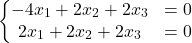A solution set is

(2)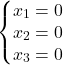This is called the trivial solution. The trivial solution to a homogeneous system is the solution with only zeros. Other solutions are called the non-trivial solutions. To see if there are other solution, we use the row reduction algorithm from the previous lesson.

(3)So, the non-trivial solution is equal to

(4)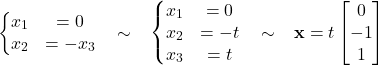In the following examples, you can see what some of the solution sets look like. If the solution set of the homogeneous set of equations has no free variables, the only solution is the trivial solution, which is the origin. If the solution set has one free variable (as in Equation 4), the solution set is a line through the origin. If the solution set has two free variables, the solution set creates a plane in the space. Take a look at the following examples.

Case 1 – The solution set of the homogeneous set of equations has no free variable.

(5)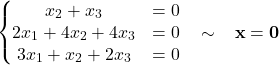Case 2 – The solution set of the homogeneous set of equations has one free variable.

(6)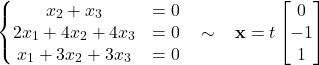Case 3 – The solution set of the homogeneous set of equations has two free variables.

(7)Inhomogeneous equations
A homogeneous system of linear equations is a system whose right hand side does not consists of only zeros. An example of a homogeneous set of equations has been given in Equation 1. An example of an inhomogeneous set of equations is given in Equation 8.

(8)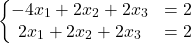Let’s first get the system in the reduced echelon form.

(9)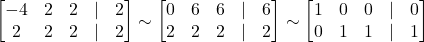The solution set is given by

(10)As we can see, a set of constants is added to the solution set. This set of constants is called the particular solution. After adding the particular solution, the graph of the solution set does not go through the origin anymore, as can be seen in Figure 4.

So, if Ap = b is consistent, then all solutions of Ax = b are given in the form x = p +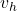(whereis the solution set of the homogeneous system), with A= 0.